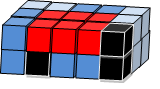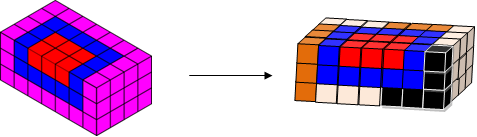#### You may also like### Just Rolling Round

P is a point on the circumference of a circle radius r which rolls, without slipping, inside a circle of radius 2r. What is the locus of P?### Coke Machine

The coke machine in college takes 50 pence pieces. It also takes a certain foreign coin of traditional design...### Just Opposite

A and C are the opposite vertices of a square ABCD, and have coordinates (a,b) and (c,d), respectively. What are the coordinates of the vertices B and D? What is the area of the square?

# Summing Squares

##### Age 14 to 16Challenge Level

We received two very detailed solutions for this problem, one by Rajeev from Fair Field Junior School and one by Furkan from Amasya Science and Arts Centre.

Both correctly concluded that in order to build the 5 by 3 by 2 cuboid using the blue square prisms they would have to split one of the square prisms. Here is Furkan's pictorial representation of how to build the cuboid.Furkan continues:

We can also surround the above cuboid by six pink prisms. Again we have to split one prism as seen in the diagram below.Rajeev noticed this pattern continued for the next 4 cuboids in the sequence.

We discovered that:

$$6 \times 1^2 = 3 \times 2 \times 1$$
$$6 \times (1^2 + 2^2) = 5 \times 3 \times 2$$
$$6 \times (1^2 + 2^2 + 3^2) = 7 \times 4 \times 3$$

Rajeev then reasoned:

The method for forming the next cuboid is adding 6 square prisms and the dimensions of the prisms are the shortest dimension of the new cuboid. For example in the $7 \times 4\times 3$ cuboid the shortest dimension is 3 so the prism is 3 by 3 by 1. To form the next cuboid surrounded say by 6 yellow square prisms the dimensions of the prisms would be 4 by 4 by 1 and the dimension of the cuboid would be 9 by 5 by 4. The formula for the number of cubes to add on is $6 \times (n+1)^2$ i.e. 6 square prisms of side n+1.

The method for finding out the number of cubes used is $(2n+1) \times (n+1) \times n$ where n is the shortest side.

I also noticed that in each cuboid the width plus the height of the cuboid equals the length. So for example in the cuboid where 12 is the shortest side the cuboid has dimensions $(2n+1) \times (n+1) \times n = 25 \times 13 \times 12$.

The dimension for the $n^{th}$ cuboid is n, n+1 and 2n+1. And for the $(n+1)^{th}$ cuboid it is n+1, n+2 and 2n+3.

So we can write the $n^{th}$ cuboid as
$$6 \times (1^2 + 2^2 + ... + n^2) = (2n+1) \times (n+1) \times n$$

Thes ideas can be used to sum the series $1^2 + 2^2 + 3^2 + ... + n^2$ quickly for any value of n using the formula:

$$1^2 + 2^2 + 3^2 + ... + n^2 = n(n+1)(2n+1)/6$$